# PLS Path Modeling in Excel: group comparison

2017-10-20

This tutorial will show you how to compare groups in a Partial Least Squares Path Modeling (PLS-PM) context in Excel using XLSTAT.

XLSTAT is the first software to offer multigroup comparison methods in the framework of PLS Path Modeling presented by Wynne Chin (2005).

In this tutorial, we present an application of multigroup tests when a path model is defined. If you are not familiar with PLS Path Modeling, please refer to the tutorial: “Creating and running a basic PLSPM project?” Two tests that allow comparing parameters between groups are included in XLSTAT:

• An adapted t test based on bootstrap standard errors. For two groups and a path coefficient beta :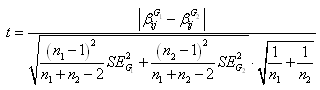Where n1 and n2 are the sizes of the groups and SE’² is the variance of coefficient beta obtained using the bootstrap sampling. This would follow a t-distribution with n1+n2-2 degrees of freedom. This approach works reasonably well if the two samples are not too non normal and the two variances are not too different from one another.
• A permutation test (randomization test): It uses a statistic S to compare parameters. This statistic is computed on the original samples and samples are permuted. The original S is compared to each permuted S in order to obtain a p-value: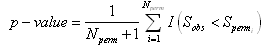This test offers a good non parametric alternative to the t test.

The hypotheses to be tested are:

• H0: parameters are not significantly different
• Ha: parameters are significantly different

More detailed explanations are available in the help of XLSTAT.

## Group comparison with XLSTAT-PLSPM

We will use the same data as in the general tutorial on how to use XLSTAT-PLSPM. It is based on the ECSI model with a sample of 250 observations. A grouping variable has been added. The new file can be downloaded here.

First of all, you have to switch to the expert display. In the XLSTAT-PLSPM toolbar, click on “XLSTAT-PLSPM options”. This dialog box appears: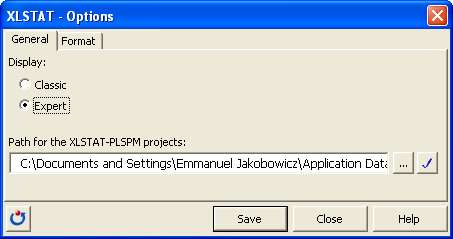Select the expert display and save your settings. As in the general tutorial build the ECSI model using the PLSPMGraph sheet and the “Path Modeling” toolbar.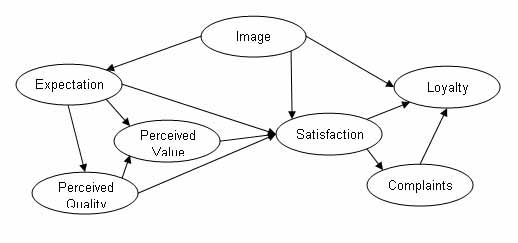Click on “define groups” in the “path modelling” toolbar. Select the “group” variable in order to define groups: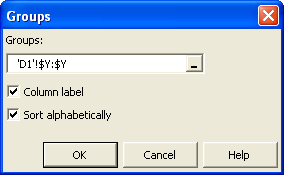Click on “run” in the “path modelling” toolbar. A new tab called “multigroup tests” has appeared: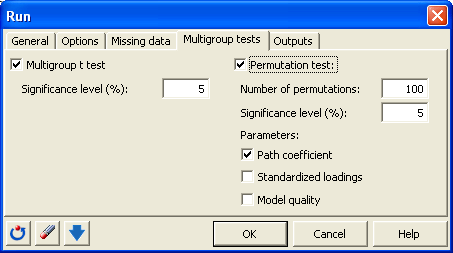Select the multigroup t test. The number of bootstrap resamples is defined in the “options” tab with the confidence intervals. Note: If confidence intervals are not estimated using bootstrap, the multigroup t test cannot be run.

Select the permutation test; adjust the number of permutation and the significance level of the test. Many parameters can be compared: path coefficients, standardized loadings and model quality indexes (communalities, redundancies and GoF).

Note: If the number of groups is larger then two, the permutation test cannot be run.

## Results and outputs of a multigroup comparison with XLSTAT-PLSPM

If groups and mutigroup tests are selected, some new outputs are available:

• Worksheet PLSPM(Group): For each group, complete results are displayed in separated worksheets.
• Worksheet PLSPM(Multigroup t test): For each path coefficient, results of the t test are summarized in a table. Each line represents a pair of groups.
• Worksheet PLSPM(Permutation test): For each type of parameter, results of the permutation test are summarized in a table.

The two first sheets can be analyzed independently using the general method described in the XLSTAT-PLSPM tutorial.

The groups have been selected using the question: How well do you think “your mobile phone provider” compares with your ideal mobile phone provider?

Results of the multigroup t test are the following: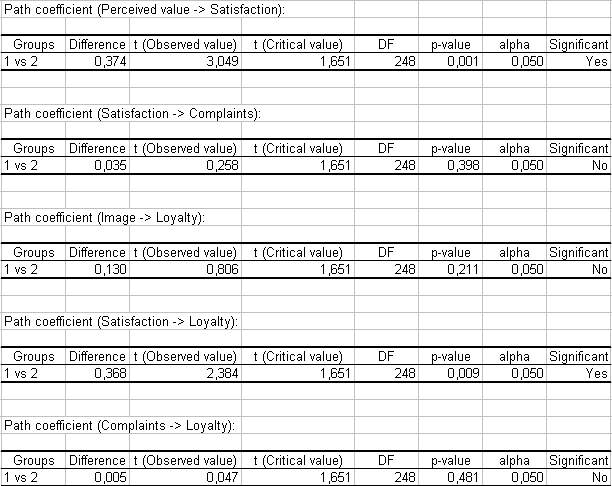Results of the multigroup permutation test are the following: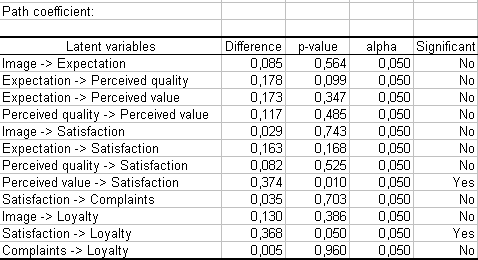These two tests results show a significant difference between the groups for two path coefficients:

• the arrow between perceived value and satisfaction
• the arrow between satisfaction and loyalty

The second one has a strategic impact in marketing studies and experts should pay a special attention to people answering negatively to the grouping question.

#### Contact our technical support team: support@xlstat.com

https://cdn.desk.com/
false
desk